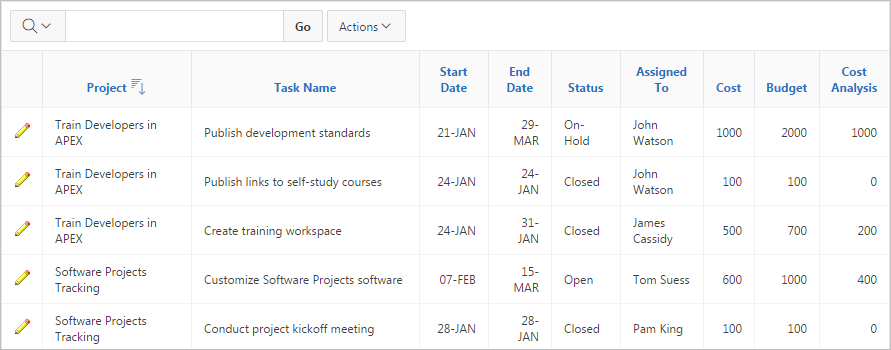## 4.6.7 Computing Columns

Add mathematical computations (for example, `NBR_HOURS/24`) or standard Oracle functions to columns.

### 4.6.7.1 Creating a Computation

Use the Compute function in the Actions, Data submenu to add a computation to a column.

To create a computation:

1. Click the Actions menu and select Data and then Compute.
The Compute dialog appears.
2. In the Compute dialog:
1. Computation - Select New Computation.
2. Column Label - Enter the name of the new column to be created.
3. Format Mask - Select an Oracle format mask to be applied to the new column. (For example, `\$5,234.10`).
3. Create the computation:
1. Columns - Select a column or alias.
2. Keypad - Select a shortcut for commonly used keys.
3. Functions - Select the appropriate function.
In the following example, a new column compares the actual cost to the budgeted amount, using the formula `I - H`, where `I` is the budgeted amount and `H` is the cost.
4. Click Apply.Description of the illustration ir_compute_eg.png

The revised report appears containing a new Cost Analysis column.

### 4.6.7.2 Deleting a Computation

Open the Compute dialog to remove a computation.

To delete a computation:

1. Click the Actions menu and select Data and then Compute.
The Compute dialog appears.
2. From Computation, select the computation.
The computation appears.
3. Click Delete.